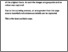# Complex boundary integral techniques for turbomachinery blading

Wood, David John (1981) Complex boundary integral techniques for turbomachinery blading. Doctoral thesis, Polytechnic of North London.Preview
Text
291927.pdf - Published Version

## Abstract / Description

Integral equation techniques are used to solve interior and exterior (including cascade) Neumann problems. The equations are in terms of the boundary values of the derivatives of the harmonic function sought. The equations are derived using the complex variables forms of Green’s theorem. They are solved numerically using low order complex polynomial approximations. The solution of problems defined on regions with corners is considered.

A complex integral representation which enables a regular function to be generated from its derivatives is derived. Approximations to the representation are given for the case when first order derivatives are known. These approximations are geared to the utilisation of the numerical solutions of the aforementioned integral equations.

Beam element solutions of stress and vibration problems require knowledge of such section properties as torsional stiffness, warping stiffness and shear centre co-ordinates. These section properties are usually expressed as integrals taken over a uniform beam’s cross section. It is shown that they can be written as boundary integrals involving the beam’s torsion and warping functions. These functions are determined using the previously mentioned integral equations together with the representation of a regular function.

The major practical application of the above techniques is to the analysis of turbomachinery blading at an early stage in the design process.

Finally, the solution of plane elastostatic problems using complex potentials is considered. A 1st Kind integral equation for the first derivative of one of the Goursat functions is introduced. The equation is shown to be derivable from a 2nd Kind equation due to Muskhelishvili. The solution of the equation is discussed in terms of a simple numerical method. Where appropriate the numerical methods described in this thesis are illustrated by examples.

Item Type: Thesis (Doctoral) uk.bl.ethos.291927 600 Technology > 620 Engineering & allied operations School of Human Sciences Maria White 19 Sep 2018 09:33 19 Sep 2018 09:33 https://repository.londonmet.ac.uk/id/eprint/3154View Item